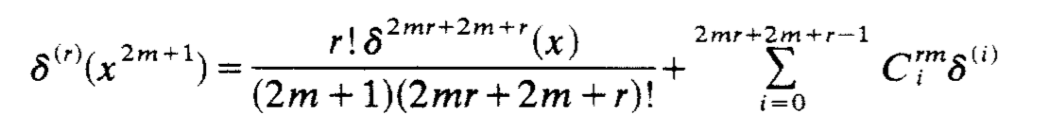# Were there attempts to express derivatives of Delta function as polynomials of Delta function?

Is seems to me that it makes sense to presume some relations between derivatives of Dirac delta functions and its powers. I wonder, whether someone proposed a similar theory?

Particularly, it could make sense that

$$\frac{\pi^3 \delta(0) ^3}{6}+\frac{\pi \delta(0) }{12}=-\pi \delta''(0)$$

In such formalism, the more "inner" peaks of the distribution (when we approximate it with a smooth function) would be represented by higher powers of $$\pi\delta(0)$$.

I wonder whether similar relations ever arised as a formal method or in any other form.

UPDATE

This paper seemingly makes an attempt, but their results are quite different. Particularly, following their paper

$$\delta''(t)=16\pi^2\delta^3(t)$$

which is different from the above expression. To my view, their approach has many weaknessess, particularly, in my view $$\delta''(0)$$ should have the opposite sign than $$\delta^3(0)$$ (which is the case if we approximate delta function with a smooth curve).

• What formalism do you have in mind in which it would be possible to multiply a distribution by itself? (or 2 different distributions with overlapping wave front sets for that matter). Apr 10 '18 at 9:11
• @Saal Hardali I encountered such relations as entirely formal links, and they seem to work for my purpose. Yet, definitely I do not know how they should be defined from the point of view of distribution theory. Each even derivative is related to a polynomial of the delta functions, the polynomials are similar to the Bernoulli polynomials (but not exactly equal). Apr 10 '18 at 9:14
• How exactly did you come up with that particular equation? And why do you only state it for $0$ in the argument? Apr 10 '18 at 9:28
• Dividing the equation by $\pi$ gives $\zeta(2)\delta(0)^3 + \zeta(-1)\delta(0) = -\delta''(0)$. Apr 10 '18 at 9:29
• @Tobias Fritz I was considering divergent integrals. This particular value represents $\int_0^\infty x^2\,dx$ (the fact this integral is equal to the right hand side can be formally derived from the Fourier transform, the left-hand side is more complicated, I was manually assessing the difference between the divergent series and the divergent integral, the divergent series can be expressed with Bernoulli polynomials of $\pi \delta(0)$. Apr 10 '18 at 9:32

There is a serious difficulty with the notion of products for distributions ; as a matter of fact Laurent Schwartz, one of the creator of Distribution Theory, wrote an article expressing the impossibility of multiplying distributions http://sites.mathdoc.fr/OCLS/pdf/OCLS_1954__21__1_0.pdf

On the other hand some simple observations are in order: just think that you want to define $\delta_0^2$, the square of the Dirac mass at 0: a natural idea would be to approximate the Dirac mass by $\rho(\frac{x}\epsilon)\frac1{\epsilon}$ with $\rho$ smooth compactly supported with integral 1 and take a look at $$\rho(\frac{x}\epsilon)^2\frac1{\epsilon^2}.$$ Considering now a test function $\phi$ say smooth and compactly supported, we may check $$\int\rho(\frac{x}\epsilon)^2\frac1{\epsilon^2}\phi(x) dx= \int\rho(t)^2\frac1{\epsilon}\phi(\epsilon t) dt\sim\frac1{\epsilon} \phi(0)\int \rho(t)^2 dt$$ if $\phi(0)\not=0$. Nonetheless there is no finite limit if $\phi(0)\not=0$, but even the factor of $1/\epsilon$ depends on $\rho$, that is on the approximation process.

A more subtle remark is due to Lars Hörmander: you may define the so-called wave-front-set of a distribution and say that if $T_1, T_2$ are distributions such that $$(x,\xi_1)\in WF T_1,\quad (x,\xi_2)\in WF T_2\Longrightarrow \xi_1+\xi_2\not=0,$$ then $T_1T_2$ makes sense. In particular you may consider $$\left(\frac{1}{x+i0}\right)^2, \quad \text{but not }\quad \delta_0^2,$$ meaning that $\lim_{\epsilon\rightarrow 0_+}\int \frac{\phi(x)}{(x+i\epsilon)^2} dx$ makes sense for any test function $\phi$. Somehow the notion of wave-front-set is providing the mathematical obstruction to a general rule of multiplication for distributions.

• But is not $$\frac1{i 0^-}=i\delta(0)$$?... May 6 '18 at 21:04

For what it's worth, a formula relating derivatives and powers of Dirac delta functions was discussed in Products and compositions with the Dirac delta function:The formula is credited to 1974 and 1977 papers by Fisher and Tewari in the journal "Mathematics Student", which I have not found accessible online.

• This is VERY interesting! But what is the second term? What does the $C$ and $\delta$ mean there? Apr 10 '18 at 14:43
• quite frankly I don't know and I hoped to learn more from the source papers in Math.Student, which I have not been able to locate; (recent issues of this journal are online, but not older issues) Apr 10 '18 at 14:55
• I am trying to insert m=0, r=1 but fail to see how this holds... Apr 10 '18 at 15:02
• Then I get $\delta'(x)=\delta(x)$. Does not hold seemingly. Apr 10 '18 at 15:06
• Maybe in the first term it is meant to be derivative as well, and not power?... Apr 10 '18 at 15:10